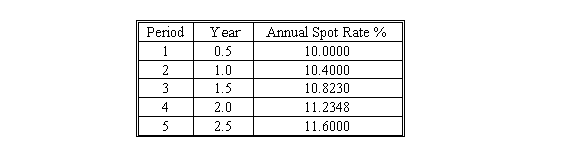### CFA Practice Question

There are 490 practice questions for this study session.

### CFA Practice Question

Given the following spot rates, the arbitrage-free value of a 5%, 2.5-year Treasury would be closest to ______.A. \$86.10
B. \$96.61
C. \$100.00

Arbitrage-free value = 2.5/(1.05)1 + 2.5/(1.052)2 + 2.5/(1.054115)3 + 2.5/(1.056174)4 + 102.5/(1.058)5= 86.10

User Comment
kalps interest each 1/2 year = 2.5 Spot rate / 2 = denominator (discount factor semi annual) Could split in to 6 different compenets if required
o123 *better off using the formula for a question like this!
Rotigga You better know how to make love to your HP-12C for a question like this.
octavianus o123: what formula are you referring to that would make this simpler?
steved333 Hey, Rotigga, I think my TI-BAII will be giving it to me rough with no rewards on this one!!!
raida any one with any ideas on how to calculate it on BA II? I doubt will have enough time to calculate it manually
thekapila on TA BA11:
FOR FIRST 3 CALCULATIONS
USE
FV = 2.5
1/Y = SPOT RATE/2
N = PERIOD MENTIONED

FOR LAST
USE FV = 100+2.5 = 102.5
SPOT RATE = 1/Y = 5.8
N = 5
PV = 86.100
jwebbs ^ so you use the length of the entire treasury as the present value all the time? or did u just get lucky?
capitalpirate gr8 work thekapila... genius!
IvanTG unfortunately you can't use a spreadsheet at the exam...
8thlegend Arbitrage-free value = 2.5/(1.05)1 + 2.5/(1.052)2 + 2.5/(1.054115)3 + 2.5/(1.056174)4 + 102.5/(1.058)5= 86.10

Where did the 2.5 come from? is it the 5% coupon 5/2?

How did they get the discount rate?
Mutsa Treasury bonds pay coupon semi annually. Annual coupon is 5% of 100 i.e 5. Semi annualis 5/2 i.e 2.5. Alos forgot and used coupon of 5%. Got a wong answer. Will keep it in mind for next time
Saxonomy I prefer...

2.5 / 1.1000^(0.5)
+ 2.5 / 1.1040^(1.0)
+ 2.5 / 1.1082^(1.5)
+ 2.5 / 1.1123^(2.0)
+ 102.5 / 1.1160^(2.5)

Just much more cleaner. Call me weird.
akils I agree with Saxonomy
jonan203 i have to figure out a way to do this on a 12c without having to write anything down.
davcer 2.38+2.25+2.13+2.0+77.3 and thats it
ascruggs92 If you know basic math you would see immediately that the stated rate and discount rate differential is too large for B. to be the answer (if maturity was 1 year away PV would be about 95). C. is obviously wrong which leaves only A.
ashish100 this is CFA, not basic math. we dont know basic math!
Logaritmus listed bond price <= price of 5 yr semi with YTM = 10% = 89.176 < B,C that easily eliminate B and C.
ZETA okay guys, the whole point of buying the notes is because we dont have the time right why on earth are these notes full of manual calculations instead of finacial calculations, I mean where is the common sense here.